# shorts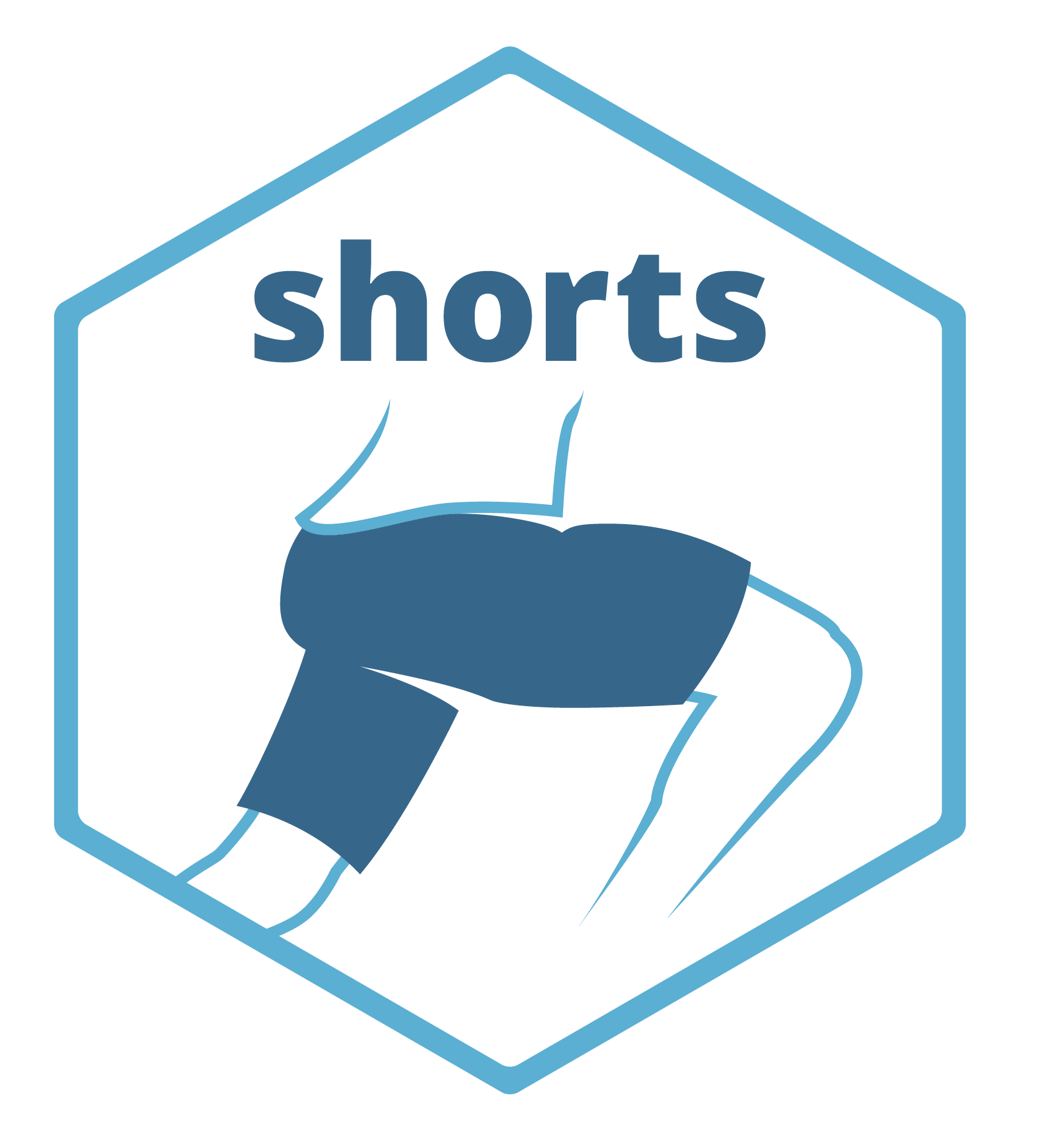This package creates short sprint (<6sec) profiles using the split times, or the radar gun data. Mono-exponential equation is used to estimate maximal sprinting speed (MSS), relative acceleration (TAU), and other parameters. These parameters can be used to predict kinematic and kinetics variables and to compare individuals.

## Installation

``````# Install from CRAN
install.packages("shorts")

# Or the development version from GitHub
# install.packages("devtools")

## Examples

{shorts} comes with two sample data sets: `split_times` and `radar_gun_data` with N=5 athletes. Let’s load them both:

``````require(shorts)
require(tidyverse)
require(knitr)

### Profiling using split times

To model sprint performance using split times, distance will be used as predictor and time as target. Since `split_times` contains data for multiple athletes, let’s extract only one athlete and model it using `shorts::model_timing_gates()` function.

``````kimberley_data <- filter(split_times, athlete == "Kimberley")

kable(kimberley_data)``````
athlete bodyweight distance time
Kimberley 55 5 1.158
Kimberley 55 10 1.893
Kimberley 55 15 2.541
Kimberley 55 20 3.149
Kimberley 55 30 4.313
Kimberley 55 40 5.444

`shorts::model_timing_gates()` returns an object with `parameters`, `model_fit`, `model` returned from `minpack.lm::nlsLM()` function and `data` used to estimate parameters. Parameters estimated using mono-exponential equation are maximal sprinting speed (MSS), and relative acceleration (TAU). Additional parameters computed from MSS and TAU are maximal acceleration (MAC) and maximal relative power (PMAX) (which is calculated as MAC*MSS/4).

``````kimberley_profile <- shorts::model_timing_gates(
distance = kimberley_data\$distance,
time = kimberley_data\$time
)

kimberley_profile
#> Estimated model parameters
#> --------------------------
#>        MSS        TAU        MAC       PMAX
#>  8.5911430  0.8113285 10.5889820 22.7428645
#>
#> Model fit estimators
#> --------------------
#>         RSE   R_squared      minErr      maxErr   maxAbsErr        RMSE
#>  0.03403413  0.99965531 -0.05293456  0.02699162  0.05293456  0.02778875
#>         MAE        MAPE
#>  0.02333341  1.19263265

summary(kimberley_profile)
#>
#> Formula: time ~ TAU * I(LambertW::W(-exp(1)^(-distance/(MSS * TAU) - 1))) +
#>     distance/MSS + TAU
#>
#> Parameters:
#>     Estimate Std. Error t value Pr(>|t|)
#> MSS  8.59114    0.12251   70.13 2.48e-07 ***
#> TAU  0.81133    0.04581   17.71 5.97e-05 ***
#> ---
#> Signif. codes:  0 '***' 0.001 '**' 0.01 '*' 0.05 '.' 0.1 ' ' 1
#>
#> Residual standard error: 0.03403 on 4 degrees of freedom
#>
#> Number of iterations to convergence: 5
#> Achieved convergence tolerance: 1.49e-08

coef(kimberley_profile)
#>        MSS        TAU        MAC       PMAX
#>  8.5911430  0.8113285 10.5889820 22.7428645``````

To return the predicted outcome (in this case time variable), use `predict()` function:

``````predict(kimberley_profile)
#>  1.210935 1.897021 2.521028 3.122008 4.299243 5.466324``````

To create a simple plot, use S3 `plot()` method:

``````plot(kimberley_profile) +
theme_bw()``````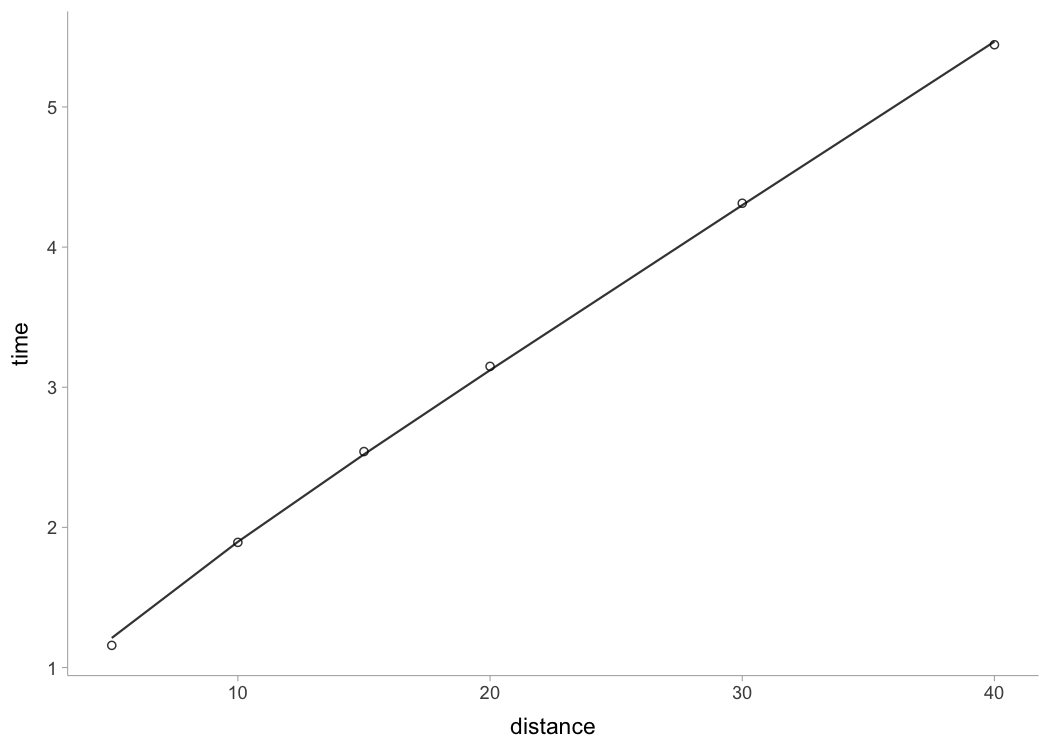If you are interested in calculating average split velocity, use `shorts::format_splits()`

``````kable(shorts::format_splits(
distance = kimberley_data\$distance,
time = kimberley_data\$time
))``````
split split_distance_start split_distance_stop split_distance split_time_start split_time_stop split_time split_mean_velocity split_mean_acceleration
1 0 5 5 0 1.158 1.158 4.317789…. 3.728660….
2 5 10 5 1.158 1.893 0.735 6.802721…. 3.380859….
3 10 15 5 1.893 2.541 0.648 7.716049…. 1.409457….
4 15 20 5 2.541 3.149 0.608 8.223684…. 0.834925….
5 20 30 10 3.149 4.313 1.164 8.591065…. 0.315619….
6 30 40 10 4.313 5.444 1.131 8.841732…. 0.221633….

Let’s plot observed vs fitted split times. For this we can use `data` returned from `shorts::model_timing_gates()` since it contains `pred_time` column.

``````ggplot(kimberley_profile\$data, aes(x = distance)) +
theme_bw() +
geom_point(aes(y = time)) +
geom_line(aes(y = pred_time)) +
xlab("Distance (m)") +
ylab("Time (s)")``````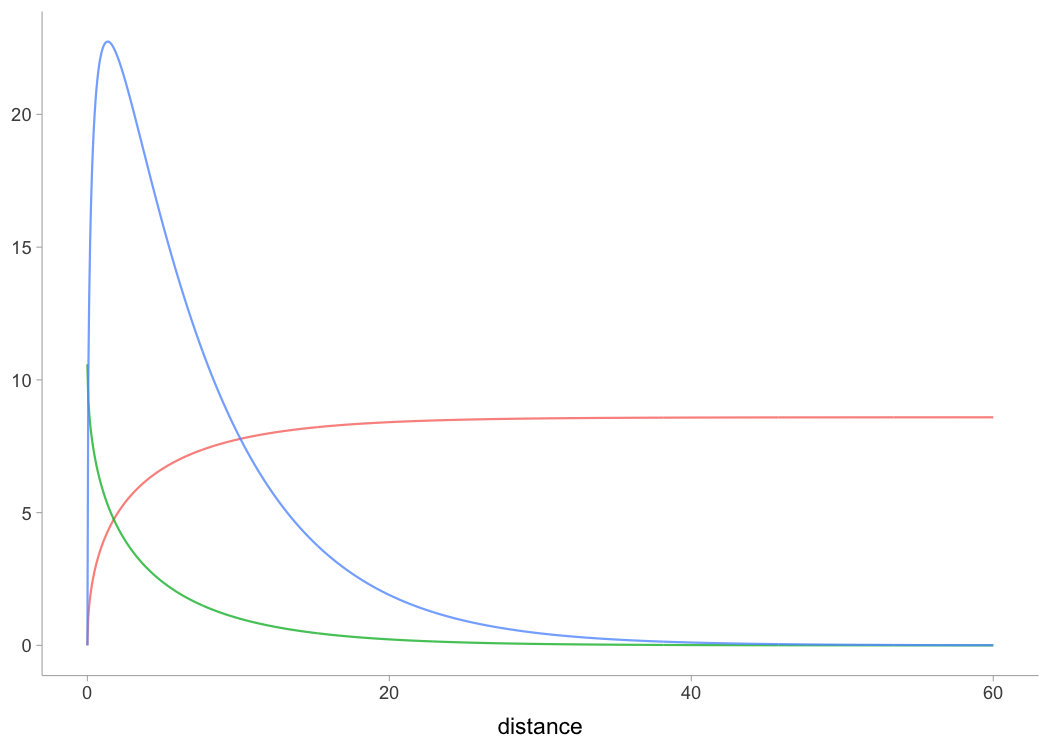To plot predicted velocity, acceleration, air resistance, force, and power over distance, use `shorts:predict_XXX()`. Please note that to calculate force, air resistance, and power, we need Kimberley’s bodymass and height (as well as other characteristics such as air pressure, temperature and wind - see `get_air_resistance()` function).

``````kimberley_bodymass <- 60 # in kilograms
kimberley_bodyheight <- 1.7 # in meters

kimberley_pred <- tibble(
distance = seq(0, 40, length.out = 1000),

# Velocity
pred_velocity = shorts::predict_velocity_at_distance(
distance,
kimberley_profile\$parameters\$MSS,
kimberley_profile\$parameters\$TAU
),

# Acceleration
pred_acceleration = shorts::predict_acceleration_at_distance(
distance,
kimberley_profile\$parameters\$MSS,
kimberley_profile\$parameters\$TAU
),

# Air resistance
pred_air_resistance = shorts::predict_air_resistance_at_distance(
distance,
kimberley_profile\$parameters\$MSS,
kimberley_profile\$parameters\$TAU,
bodymass = kimberley_bodymass,
bodyheight = kimberley_bodyheight
),

# Force
pred_force = shorts::predict_force_at_distance(
distance,
kimberley_profile\$parameters\$MSS,
kimberley_profile\$parameters\$TAU,
bodymass = kimberley_bodymass,
bodyheight = kimberley_bodyheight
),

# Power
pred_power = shorts::predict_power_at_distance(
distance,
kimberley_profile\$parameters\$MSS,
kimberley_profile\$parameters\$TAU,
bodymass = kimberley_bodymass,
bodyheight = kimberley_bodyheight
),
)

# Convert to long
kimberley_pred <- gather(kimberley_pred, "metric", "value", -distance)

ggplot(kimberley_pred, aes(x = distance, y = value)) +
theme_bw() +
geom_line() +
facet_wrap(~metric, scales = "free_y") +
xlab("Distance (m)") +
ylab(NULL)``````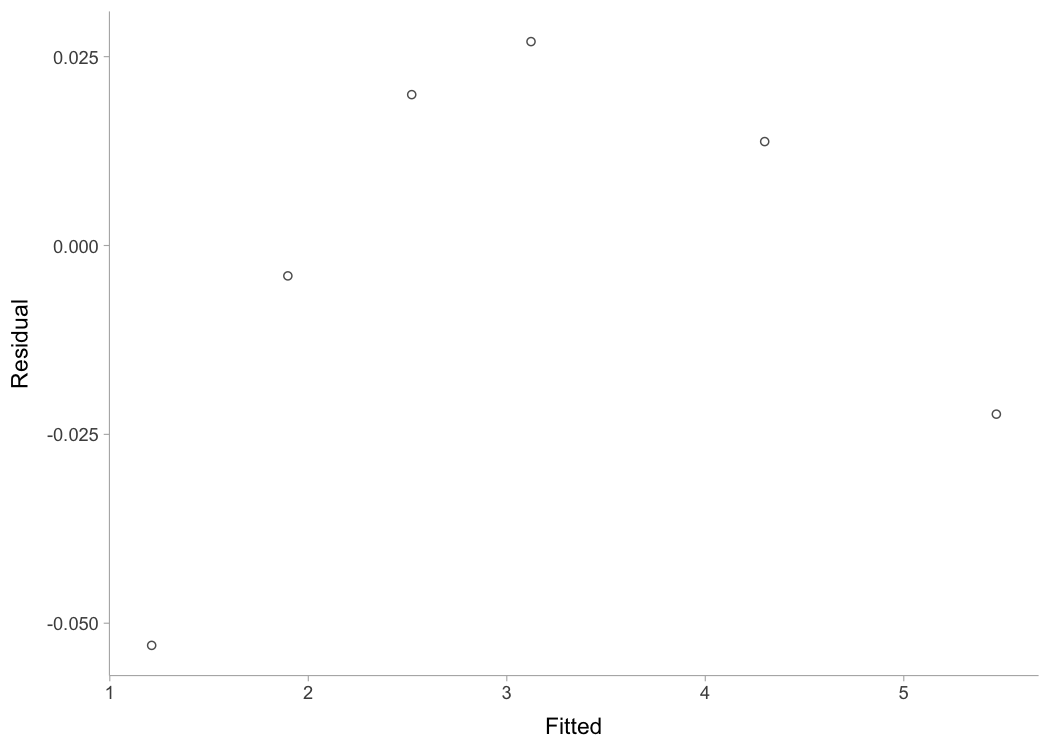To do prediction simpler, use `shorts::predict_kinematics()` function. This will provide kinetics and kinematics for 0-6s sprint using 100Hz.

``````predicted_kinematics <- predict_kinematics(
kimberley_profile,
bodymass = kimberley_bodymass,
bodyheight = kimberley_bodyheight
)

time distance velocity acceleration bodymass inertia resistance net_horizontal_force air_resistance horizontal_force horizontal_force_relative vertical_force resultant_force resultant_force_relative power relative_power RF force_angle
0.00 0.0000000 0.0000000 10.588982 60 0 0 635.3389 0.0000000 635.3389 10.588982 588.6 866.0863 14.43477 0.00000 0.000000 0.7335746 42.81309
0.01 0.0005273 0.1052399 10.459269 60 0 0 627.5561 0.0026620 627.5588 10.459313 588.6 860.3953 14.33992 66.04424 1.100737 0.7293843 43.16520
0.02 0.0021005 0.2091907 10.331145 60 0 0 619.8687 0.0105181 619.8792 10.331320 588.6 854.8100 14.24683 129.67295 2.161216 0.7251660 43.51734
0.03 0.0047068 0.3118680 10.204590 60 0 0 612.2754 0.0233774 612.2988 10.204980 588.6 849.3290 14.15548 190.95643 3.182607 0.7209206 43.86946
0.04 0.0083337 0.4132876 10.079586 60 0 0 604.7751 0.0410543 604.8162 10.080270 588.6 843.9506 14.06584 249.96306 4.166051 0.7166488 44.22151
0.05 0.0129685 0.5134649 9.956113 60 0 0 597.3668 0.0633688 597.4301 9.957169 588.6 838.6732 13.97789 306.75938 5.112656 0.7123515 44.57343

To get model residuals, use `residuals()` function:

``````residuals(kimberley_profile)
#>  -0.052934560 -0.004021206  0.019971712  0.026991617  0.013756878
#>  -0.022324483``````

Package {shorts} comes with `find_XXX()` family of functions that allow finding peak power and it’s location, as well as critical distance over which velocity, acceleration, or power drops below certain threshold:

``````# Peak power and location
shorts::find_peak_power_distance(
MSS = kimberley_profile\$parameters\$MSS,
MAC = kimberley_profile\$parameters\$MAC,
bodymass = kimberley_bodymass,
bodyheight = kimberley_bodyheight
)
#> \$peak_power
#>  1384.248
#>
#> \$distance
#>  1.424183

# Distance over which power is over 80%
shorts::find_power_critical_distance(
MSS = kimberley_profile\$parameters\$MSS,
MAC = kimberley_profile\$parameters\$MAC,
bodymass = kimberley_bodymass,
bodyheight = kimberley_bodyheight,
percent = 0.8
)
#> \$lower
#>  0.3421958
#>
#> \$upper
#>  4.26947

# Distance over which acceleration is under 50%
shorts::find_acceleration_critical_distance(
MSS = kimberley_profile\$parameters\$MSS,
MAC = kimberley_profile\$parameters\$MAC,
percent = 0.5
)
#>  1.34628

# Distance over which velocity is over 95%
shorts::find_velocity_critical_distance(
MSS = kimberley_profile\$parameters\$MSS,
MAC = kimberley_profile\$parameters\$MAC,
percent = 0.95
)
#>  14.25923``````

### Profiling using radar gun data

The radar gun data is modeled using measured velocity as target variable and time as predictor. Individual analysis is performed using `shorts::model_radar_gun()` function. Let’s do analysis for Jim:

``````jim_data <- filter(radar_gun_data, athlete == "Jim")

time = jim_data\$time,
velocity = jim_data\$velocity
)

jim_profile
#> Estimated model parameters
#> --------------------------
#>          MSS          TAU          MAC         PMAX           TC
#> 7.998011e+00 8.887955e-01 8.998708e+00 1.799294e+01 1.103989e-04
#>
#> Model fit estimators
#> --------------------
#>         RSE   R_squared      minErr      maxErr   maxAbsErr        RMSE
#>  0.05062927  0.99924409 -0.16404520  0.15112314  0.16404520  0.05050254
#>         MAE        MAPE
#>  0.03927236         Inf

summary(jim_profile)
#>
#> Formula: velocity ~ MSS * (1 - exp(1)^(-(time + TC)/TAU))
#>
#> Parameters:
#>      Estimate Std. Error t value Pr(>|t|)
#> MSS 7.9980114  0.0031934 2504.54   <2e-16 ***
#> TAU 0.8887955  0.0021794  407.81   <2e-16 ***
#> TC  0.0001104  0.0012283    0.09    0.928
#> ---
#> Signif. codes:  0 '***' 0.001 '**' 0.01 '*' 0.05 '.' 0.1 ' ' 1
#>
#> Residual standard error: 0.05063 on 597 degrees of freedom
#>
#> Number of iterations to convergence: 6
#> Achieved convergence tolerance: 1.49e-08

plot(jim_profile) +
theme_bw()``````The object returned from `shorts::model_radar_gun()` is same as object returned from `shorts::model_timing_gates()`. Let’s plot Jim’s measured velocity and predicted velocity:

``````ggplot(jim_profile\$data, aes(x = time)) +
theme_bw() +
geom_line(aes(y = velocity), alpha = 0.5) +
geom_line(aes(y = pred_velocity), color = "red", alpha = 0.5) +
xlab("Time (s)") +
ylab("Velocity (m/s)")``````Rather than estimating MSS, `shorts::model_radar_gun()` function allows you to utilize peak velocity observed in the data as MSS. This is done by setting the `use_observed_MSS` parameter to `TRUE`:

``````jim_profile <- shorts::model_radar_gun(
time = jim_data\$time,
velocity = jim_data\$velocity,
use_observed_MSS = TRUE
)

jim_profile
#> Estimated model parameters
#> --------------------------
#>         MSS         TAU         MAC        PMAX          TC
#>  8.09500000  0.93279456  8.67822382 17.56255546  0.01117667
#>
#> Model fit estimators
#> --------------------
#>         RSE   R_squared      minErr      maxErr   maxAbsErr        RMSE
#>  0.08004636  0.99875693 -0.22869210  0.18253899  0.22869210  0.07984600
#>         MAE        MAPE
#>  0.06431869         Inf

summary(jim_profile)
#>
#> Formula: velocity ~ MSS * (1 - exp(1)^(-(time + TC)/TAU))
#>
#> Parameters:
#>     Estimate Std. Error  t value Pr(>|t|)
#> MSS 8.095000   0.005209 1554.099  < 2e-16 ***
#> TAU 0.932795   0.003605  258.739  < 2e-16 ***
#> TC  0.011177   0.002025    5.519  5.1e-08 ***
#> ---
#> Signif. codes:  0 '***' 0.001 '**' 0.01 '*' 0.05 '.' 0.1 ' ' 1
#>
#> Residual standard error: 0.08005 on 597 degrees of freedom
#>
#> Number of iterations to convergence: 5
#> Achieved convergence tolerance: 1.49e-08``````

### Profiling using tether devices

Some tether devices provide data out in a velocity-at-distance format. In this case, velocity is the outcome variable and distance is the predictor. To estimate sprint profiles from tether data, use `shorts::model_tether()` function:

``````distance <- c(5, 10, 20, 30, 40)

velocity <- predict_velocity_at_distance(distance, MSS = 10, MAC = 8)

m1 <- model_tether(distance = distance, velocity = velocity)

df <- data.frame(
distance = distance,
obs_velocity = velocity
)

plot(m1) +
geom_point(data = df, aes(x = distance, y = obs_velocity))``````Setting `use_observed_MSS` parameter to `TRUE` in the `shorts::model_tether()` function also allows you to use observed peak velocity as MSS.

In the case when distance is not centered at zero, use `shorts::model_tether_DC()` which also estimated the distance correction (DC) parameter, serving as model intercept:

``````distance <- c(5, 10, 20, 30, 40)

velocity <- predict_velocity_at_distance(distance - 0.5, MSS = 10, MAC = 8)

m1 <- model_tether_DC(distance = distance, velocity = velocity)
m1
#> Estimated model parameters
#> --------------------------
#>   MSS   TAU   MAC  PMAX    DC
#> 10.00  1.25  8.00 20.00 -0.50
#>
#> Model fit estimators
#> --------------------
#>          RSE    R_squared       minErr       maxErr    maxAbsErr         RMSE
#> 1.538370e-15 1.000000e+00 0.000000e+00 1.776357e-15 1.776357e-15 9.729507e-16
#>          MAE         MAPE
#> 7.105427e-16 8.773026e-15``````

### Force-Velocity Profiling

To estimate Force-Velocity Profile (FVP) using approach by Samozino et al. (2016, 2022) use `shorts::make_FV_profile()`:

``````kimberley_fv <- shorts::make_FV_profile(
MSS = kimberley_profile\$parameters\$MSS,
MAC = kimberley_profile\$parameters\$MAC,
# These are needed to estimate air resistance
bodymass = kimberley_bodymass,
bodyheight = kimberley_bodyheight
)

kimberley_fv
#> \$bodymass
#>  60
#>
#> \$F0
#>  635.3389
#>
#> \$F0_rel
#>  10.58898
#>
#> \$V0
#>  8.845438
#>
#> \$Pmax
#>  1404.963
#>
#> \$Pmax_rel
#>  23.41605
#>
#> \$FV_slope
#>  -1.197112``````

To convert back to Acceleration-Velocity Profile (AVP), use:

``````kimberley_avp <- shorts::convert_FV(
F0 = kimberley_fv\$F0,
V0 = kimberley_fv\$V0,
bodymass = kimberley_bodymass,
bodyheight = kimberley_bodyheight
)

kimberley_avp
#> \$MSS
#>  8.591143
#>
#> \$MAC
#>  10.58898``````

{shorts} package also allows utilizing external load in estimating FVP, as well as using FVP parameters to predict kinematic and kinetic variables. External load is represented either with additional inertia (i.e., weight vest), horizontal resistance (i.e., tether device that create additional resistance or help, or a hill sprinting), or both (i.e., a sled, which have both inertia and resistance due to friction forces).

Let’s see how theoretical model, assuming FVP is determinant of performance (which I do not agree with, BTW), predicts changes in sprint characteristics (i.e., MSS and MAC) under different external load conditions and magnitudes using Kimberley’s estimated FVP:

``````loads_df <- rbind(
tibble(type = "Weight vest", magnitude = seq(0, 20, length.out = 100), inertia = magnitude, resistance = 0),
tibble(type = "Tether", magnitude = seq(-50, 200, length.out = 100), inertia = 0, resistance = magnitude),
tibble(type = "Sled", magnitude = seq(0, 40, length.out = 100), inertia = magnitude, resistance = magnitude * 9.81 * 0.4)
) %>%
mutate(
data.frame(shorts::convert_FV(
F0 = kimberley_fv\$F0,
V0 = kimberley_fv\$V0,
bodymass = kimberley_bodymass,
bodyheight = kimberley_bodyheight,
inertia = inertia,
resistance = resistance
))
)

pivot_longer(cols = c(MSS, MAC), names_to = "parameter") %>%
ggplot(aes(x = magnitude, y = value, color = parameter)) +
theme_bw() +
geom_vline(xintercept = 0, linetype = "dotted") +
geom_line() +
facet_wrap(~type, scales = "free_x") +
ylab(NULL)``````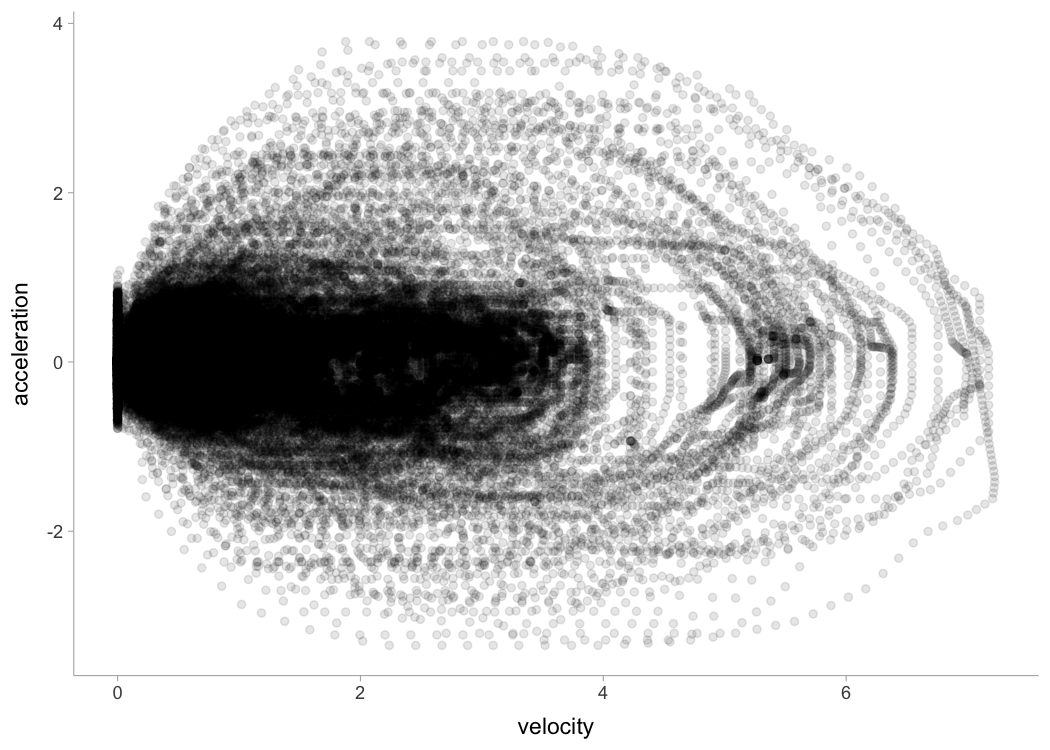Following figure depicts the effect on split times under different load types and magnitudes, assuming FVP to be determinant of performance (i.e., causal mechanism):

``````dist_df <- expand_grid(
distance = c(5, 10, 20, 30, 40)
) %>%
mutate(
time = predict_time_at_distance(distance, MSS, MAC),
distance = factor(
paste0(distance, "m"), levels = c("5m", "10m", "20m", "30m", "40m"))
)

dist_df %>%
ggplot(aes(x = magnitude, y = time, color = distance)) +
theme_bw() +
geom_vline(xintercept = 0, linetype = "dotted") +
geom_line() +
facet_wrap(~type, scales = "free_x") +
ylab("Time (s)")``````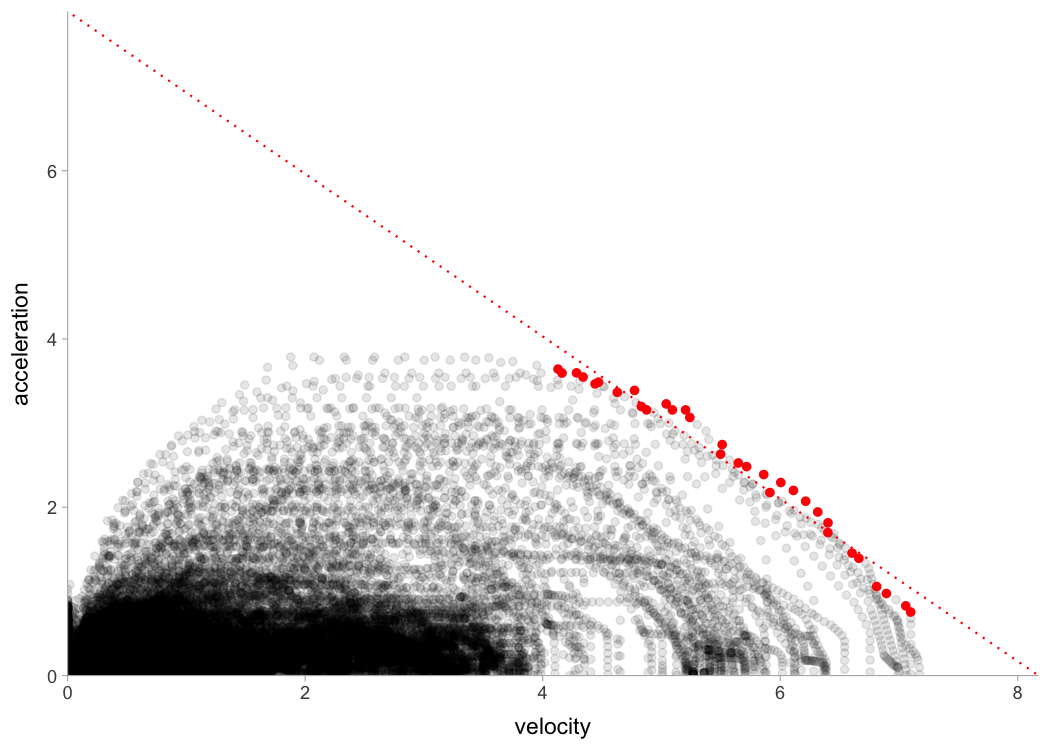One can use external resistance when predicting force or power:

``````shorts::predict_force_at_time(
time = 0.5,
MSS = 9,
MAC = 7,
bodymass = 75,
inertia = 20,
resistance = 50)
#>  503.0126

shorts::predict_power_at_time(
time = 0.5,
MSS = 9,
MAC = 7,
bodymass = 75,
inertia = 20,
resistance = 50)
#>  1458.593

shorts::predict_time_at_distance_FV(
distance = 10,
F0 = 750,
V0 = 8,
bodymass = 75,
inertia = 20,
resistance = 50)
#>  2.259444``````

External resistances can also be utilized in the Optimization functions, covered later.

### Using corrections

You have probably noticed that estimated MSS and TAU were a bit too high for splits data. Biased estimates are due to differences in starting positions and timing triggering methods for certain measurement approaches (e.g. starting behind first timing gate, or allowing for body rocking).

Here I will provide quick summary. Often, this bias in estimates is dealt with by using heuristic rule of thumb of adding time correction (`time_correction`) to split times (e.g. from 0.3-0.5sec; see more in Haugen et al., 2012). To do this, just add time correction to time split:

``````kimberley_profile_fixed_TC <- shorts::model_timing_gates(
distance = kimberley_data\$distance,
time = kimberley_data\$time + 0.3
)

kimberley_profile_fixed_TC
#> Estimated model parameters
#> --------------------------
#>       MSS       TAU       MAC      PMAX
#>  9.127769  1.377624  6.625731 15.119536
#>
#> Model fit estimators
#> --------------------
#>          RSE    R_squared       minErr       maxErr    maxAbsErr         RMSE
#>  0.009969260  0.999971375 -0.007689947  0.016398636  0.016398636  0.008139867
#>          MAE         MAPE
#>  0.006393853  0.285701748

summary(kimberley_profile_fixed_TC)
#>
#> Formula: time ~ TAU * I(LambertW::W(-exp(1)^(-distance/(MSS * TAU) - 1))) +
#>     distance/MSS + TAU
#>
#> Parameters:
#>     Estimate Std. Error t value Pr(>|t|)
#> MSS  9.12777    0.05355  170.44 7.11e-09 ***
#> TAU  1.37762    0.02131   64.66 3.43e-07 ***
#> ---
#> Signif. codes:  0 '***' 0.001 '**' 0.01 '*' 0.05 '.' 0.1 ' ' 1
#>
#> Residual standard error: 0.009969 on 4 degrees of freedom
#>
#> Number of iterations to convergence: 5
#> Achieved convergence tolerance: 1.49e-08

coef(kimberley_profile_fixed_TC)
#>       MSS       TAU       MAC      PMAX
#>  9.127769  1.377624  6.625731 15.119536``````

Instead of providing for `TC`, this parameter can be estimated using `shorts::model_timing_gates_TC()`.

``````kimberley_profile_TC <- shorts::model_timing_gates_TC(
distance = kimberley_data\$distance,
time = kimberley_data\$time
)

kimberley_profile_TC
#> Estimated model parameters
#> --------------------------
#>        MSS        TAU        MAC       PMAX         TC
#>  8.9748354  1.2348566  7.2679173 16.3070903  0.2346537
#>
#> Model fit estimators
#> --------------------
#>           RSE     R_squared        minErr        maxErr     maxAbsErr
#>  0.0011290466  0.9999996942 -0.0011807345  0.0012094658  0.0012094658
#>          RMSE           MAE          MAPE
#>  0.0007983565  0.0006586034  0.0282353187``````

Instead of estimating `TC`, {shorts} package features a method of estimating flying start distance (`FD`):

``````kimberley_profile_FD <- shorts::model_timing_gates_FD(
distance = kimberley_data\$distance,
time = kimberley_data\$time
)

kimberley_profile_FD
#> Estimated model parameters
#> --------------------------
#>        MSS        TAU        MAC       PMAX         FD
#>  9.0026808  1.2877008  6.9912831 15.7350726  0.3015635
#>
#> Model fit estimators
#> --------------------
#>           RSE     R_squared        minErr        maxErr     maxAbsErr
#>  0.0003901336  0.9999999635 -0.0004036159  0.0004557031  0.0004557031
#>          RMSE           MAE          MAPE
#>  0.0002758661  0.0002367539  0.0078291094``````

If you want to use fixed `FD` parameter (e.g., when you know what is the flying distance), in a similar vein of using fixed `TC` correction, use:

``````kimberley_profile_fixed_FD <- shorts::model_timing_gates_FD(
distance = kimberley_data\$distance,
time = kimberley_data\$time,
FD = 0.5
)

kimberley_profile_fixed_FD
#> Estimated model parameters
#> --------------------------
#>       MSS       TAU       MAC      PMAX        FD
#>  9.178464  1.472935  6.231413 14.298700  0.500000
#>
#> Model fit estimators
#> --------------------
#>          RSE    R_squared       minErr       maxErr    maxAbsErr         RMSE
#>  0.011228028  0.999973897 -0.007903690  0.015461987  0.015461987  0.007939415
#>          MAE         MAPE
#>  0.006718969  0.349905006``````

### Cross-Validation (CV)

`model_timing_gates_()` family of functions come with LOOCV feature that is performed by setting the function parameter `LOOCV = TRUE`. This feature is very useful for checking model parameters robustness and model predictions on unseen data. LOOCV involve iterative model building and testing by removing observation one by one and making predictions for them. Let’s use Kimberley again, but this time perform LOOCV:

``````kimberley_profile_LOOCV <- shorts::model_timing_gates(
distance = kimberley_data\$distance,
time = kimberley_data\$time,
LOOCV = TRUE
)

kimberley_profile_LOOCV
#> Estimated model parameters
#> --------------------------
#>        MSS        TAU        MAC       PMAX
#>  8.5911430  0.8113285 10.5889820 22.7428645
#>
#> Model fit estimators
#> --------------------
#>         RSE   R_squared      minErr      maxErr   maxAbsErr        RMSE
#>  0.03403413  0.99965531 -0.05293456  0.02699162  0.05293456  0.02778875
#>         MAE        MAPE
#>  0.02333341  1.19263265
#>
#>
#> Cross-Validation
#> ------------------------------
#> Parameters:
#> # A tibble: 6 × 4
#>     MSS   TAU   MAC  PMAX
#>   <dbl> <dbl> <dbl> <dbl>
#> 1  8.69 0.856  10.2  22.1
#> 2  8.60 0.815  10.5  22.7
#> 3  8.56 0.795  10.8  23.0
#> 4  8.57 0.797  10.8  23.0
#> 5  8.61 0.813  10.6  22.8
#> 6  8.39 0.760  11.1  23.2
#>
#> Testing model fit:
#>         RSE   R_squared      minErr      maxErr   maxAbsErr        RMSE
#>          NA  0.99901083 -0.08009035  0.03444978  0.08009035  0.04742764
#>         MAE        MAPE
#>  0.03923868  1.72270378``````

Box-plot is suitable method for plotting estimated parameters:

``````LOOCV_parameters <- gather(kimberley_profile_LOOCV\$CV\$parameters)

ggplot(LOOCV_parameters, aes(y = value)) +
theme_bw() +
geom_boxplot() +
facet_wrap(~key, scales = "free") +
ylab(NULL) +
theme(axis.ticks.x = element_blank(), axis.text.x = element_blank())``````Let’s plot model LOOCV predictions and training (when using all data set) predictions against observed performance:

``````kimberley_data <- kimberley_data %>%
mutate(
pred_time = predict(kimberley_profile_LOOCV),
LOOCV_time = kimberley_profile_LOOCV\$CV\$data\$pred_time
)

ggplot(kimberley_data, aes(x = distance)) +
theme_bw() +
geom_point(aes(y = time)) +
geom_line(aes(y = pred_time), color = "black") +
geom_line(aes(y = LOOCV_time), color = "red") +
xlab("Distance (m)") +
ylab("Time (s)")``````Let’s plot predicted velocity using LOOCV estimate parameters to check robustness of the model predictions:

``````plot_data <- kimberley_profile_LOOCV\$CV\$parameters %>%
mutate(LOOCV = row_number())

plot_data <- expand_grid(
data.frame(time = seq(0, 6, length.out = 100)),
plot_data
) %>%
mutate(
LOOCV_velocity = predict_velocity_at_time(
time = time,
MSS = MSS,
MAC = MAC
),
velocity = predict_velocity_at_time(
time = time,
MSS = kimberley_profile_LOOCV\$parameters\$MSS,
MAC = kimberley_profile_LOOCV\$parameters\$MAC
)
)

ggplot(plot_data, aes(x = time, y = LOOCV_velocity, group = LOOCV)) +
theme_bw() +
geom_line(alpha = 0.8) +
geom_line(aes(y = velocity), color = "red", size = 0.5) +
xlab("Time (sec)") +
ylab("Velocity (m/s)")``````Cross-validation implemented in `model_radar_gun()` function involves using n-folds, set by using `CV=` parameter:

``````jim_profile_CV <- shorts::model_radar_gun(
time = jim_data\$time,
velocity = jim_data\$velocity,
CV = 10
)

jim_profile_CV
#> Estimated model parameters
#> --------------------------
#>          MSS          TAU          MAC         PMAX           TC
#> 7.998011e+00 8.887955e-01 8.998708e+00 1.799294e+01 1.103989e-04
#>
#> Model fit estimators
#> --------------------
#>         RSE   R_squared      minErr      maxErr   maxAbsErr        RMSE
#>  0.05062927  0.99924409 -0.16404520  0.15112314  0.16404520  0.05050254
#>         MAE        MAPE
#>  0.03927236         Inf
#>
#>
#> Cross-Validation
#> ------------------------------
#> Parameters:
#> # A tibble: 10 × 5
#>      MSS   TAU   MAC  PMAX         TC
#>    <dbl> <dbl> <dbl> <dbl>      <dbl>
#>  1  8.00 0.888  9.00  18.0  0.0000839
#>  2  8.00 0.889  8.99  18.0  0.000457
#>  3  8.00 0.889  9.00  18.0 -0.000151
#>  4  8.00 0.889  9.00  18.0 -0.0000337
#>  5  8.00 0.888  9.00  18.0  0.000124
#>  6  8.00 0.889  9.00  18.0  0.000107
#>  7  8.00 0.888  9.01  18.0 -0.0000673
#>  8  8.00 0.889  9.00  18.0  0.0000695
#>  9  8.00 0.889  8.99  18.0  0.000263
#> 10  8.00 0.889  9.00  18.0  0.000259
#>
#> Testing model fit:
#>         RSE   R_squared      minErr      maxErr   maxAbsErr        RMSE
#>          NA  0.99923997 -0.16426599  0.15245264  0.16426599  0.05063981
#>         MAE        MAPE
#>  0.03940131         Inf``````

### Optimization

Using the method outlined in Samozino et al (2022), one can find the optimal profiles, as well as the profile imbalance (compared to the optimal), for both sprint profiles (i.e., MSS and MAC) and Force-Velocity (FV). In addition to this, one can probe the profiles (i.e., increase V0/F0 or MSS/MAC for say 2.5% to check which improvement yield more improvement in sprint time). The following graph depicts estimate profile imbalances. Note that >100% is velocity deficit (i.e., increasing velocity; MSS or V0; will yield more improvement in sprint times), while <100% is force deficit.

``````MSS <- 10
MAC <- 8
bodymass <- 75

fv <- make_FV_profile(MSS, MAC, bodymass)

opt_df <- tibble(
dist = seq(5, 50, by = 5)
) %>%
mutate(
`Sprint Profile` = optimal_MSS_MAC(
distance = dist,
MSS,
MAC
)[["profile_imb"]],
`FV Profile` = optimal_FV(
distance = dist,
fv\$F0,
fv\$V0,
bodymass
)[["profile_imb"]],
`FV Profile (PeakPower)` = optimal_FV(
distance = dist,
fv\$F0,
fv\$V0,
bodymass,
method = "peak"
)[["profile_imb"]],
`Probe FV` = probe_FV(
distance = dist,
fv\$F0,
fv\$V0,
bodymass
)[["profile_imb"]],
`Probe MSS/MAC` = probe_MSS_MAC(
distance = dist,
MSS,
MAC
)[["profile_imb"]]
) %>%
pivot_longer(-dist, names_to = "profile")

opt_dist <- tibble(
`Sprint Profile` = find_optimal_distance(
MSS,
MAC,
optimal_func = optimal_MSS_MAC
),
`FV Profile` = find_optimal_distance(
fv\$F0,
fv\$V0,
bodymass,
optimal_func = optimal_FV
),
`FV Profile (PeakPower)` = find_optimal_distance(
fv\$F0,
fv\$V0,
bodymass,
optimal_func = optimal_FV,
method = "peak"
),
`Probe FV` = find_optimal_distance(
fv\$F0,
fv\$V0,
bodymass,
optimal_func = probe_FV
),
`Probe MSS/MAC` = find_optimal_distance(
MSS,
MAC,
optimal_func = probe_MSS_MAC
)
) %>%
pivot_longer(cols = 1:5, names_to = "profile")

ggplot(opt_df, aes(x = dist, y = value, color = profile)) +
theme_bw() +
geom_hline(yintercept = 100, linetype = "dashed", alpha = 0.6) +
geom_line() +
geom_point(data = opt_dist, aes(x = value, y = 100), size = 2) +
xlab("Distance (m)") +
ylab("Profile imbalance")``````## Publications

1. Jovanović, M., Vescovi, J.D. (2022). {shorts}: An R Package for Modeling Short Sprints. International Journal of Strength and Conditioning, 2(1). https://doi.org/10.47206/ijsc.v2i1.74

2. Jovanović M. (2023). Bias in estimated short sprint profiles using timing gates due to the flying start: simulation study and proposed solutions. Computer Methods in Biomechanics and Biomedical Engineering:1–11. https://doi.org/10.1080/10255842.2023.2170713

3. Vescovi, JD and Jovanović, M. (2021). Sprint Mechanical Characteristics of Female Soccer Players: A Retrospective Pilot Study to Examine a Novel Approach for Correction of Timing Gate Starts. Front Sports Act Living 3: 629694, 2021. https://doi.org/10.3389/fspor.2021.629694

## Citation

To cite {shorts}, please use the following command to get the BibTex entry:

``citation("shorts")``

Chelly SM, Denis C. 2001. Leg power and hopping stiffness: relationship with sprint running performance: Medicine and Science in Sports and Exercise:326–333. DOI: 10.1097/00005768-200102000-00024.

Clark KP, Rieger RH, Bruno RF, Stearne DJ. 2017. The NFL Combine 40-Yard Dash: How Important is Maximum Velocity? Journal of Strength and Conditioning Research:1. DOI: 10.1519/JSC.0000000000002081.

Furusawa K, Hill AV, and Parkinson JL. The dynamics of” sprint” running. Proceedings of the Royal Society of London. Series B, Containing Papers of a Biological Character 102 (713): 29-42, 1927

Greene PR. 1986. Predicting sprint dynamics from maximum-velocity measurements. Mathematical Biosciences 80:1–18. DOI: 10.1016/0025-5564(86)90063-5.

Haugen TA, Tønnessen E, Seiler SK. 2012. The Difference Is in the Start: Impact of Timing and Start Procedure on Sprint Running Performance: Journal of Strength and Conditioning Research 26:473–479. DOI: 10.1519/JSC.0b013e318226030b.

Samozino P, Rabita G, Dorel S, Slawinski J, Peyrot N, Saez de Villarreal E, Morin J-B. 2016. A simple method for measuring power, force, velocity properties, and mechanical effectiveness in sprint running: Simple method to compute sprint mechanics. Scandinavian Journal of Medicine & Science in Sports 26:648–658. DOI: 10.1111/sms.12490.

Samozino P. 2018. A Simple Method for Measuring Force, Velocity and Power Capabilities and Mechanical Effectiveness During Sprint Running. In: Morin J-B, Samozino P eds. Biomechanics of Training and Testing. Cham: Springer International Publishing, 237–267. DOI: 10.1007/978-3-319-05633-3_11.

Samozino P, Peyrot N, Edouard P, Nagahara R, Jimenez‐Reyes P, Vanwanseele B, Morin J. 2022. Optimal mechanical force‐velocity profile for sprint acceleration performance.Scandinavian Journal of Medicine & Science in Sports 32:559–575. DOI: 10.1111/sms.14097.